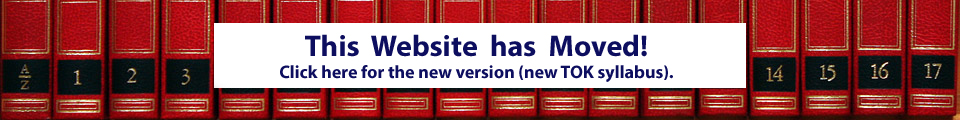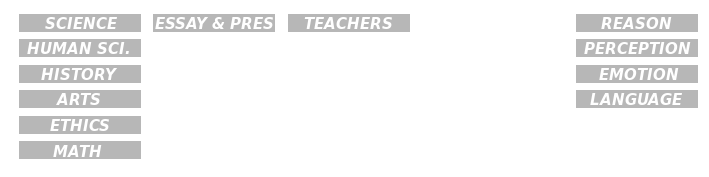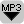## What are Formal Systems?Introduced here: the MIU puzzle as an example of a formal system. A formal system is composed of axioms, to which rules of inference are applied to produce theorems to which the rules can be applied again. Confused? Try to MIU puzzle yourself – it’s fun!

The MU Puzzle is an example of a formal system. The objective of the MU Puzzle is to try to reach the string MU starting from MI, using only these four rules:

• Rule 1: xI ? xIU. If there is an I at the end of the string of letters, then you can add a U. For example if your string is MI then you can change it into MIU. You can only add a U if the last letter is an I.
• Rule 2: Mx ? Mxx. You can double any string that follows the M. So if your string is MIU then you can double the IU after the M. You will then get MIUIU. We have doubled the IU.